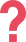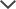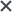I got the ACCUPLACER pack. I found TestPrep-Online’s preparation pack very helpful. The questions and study guides are great. I found that the explanations and solving tips really helped me to learn the material and improve my score.
Nicole
My 3rd grade daughter was recommended to take the CogAT. We loved the explanations as they helped her to understand the material. The packs are a helpful tool and less expensive than a private tutor.
Aminata
We got the CogAT practice tests for our daughter. She nailed the test and I would totally recommend TestPrep-Online to other parents.
Jonathan
We used the CogAT practice tests and I would definitely recommend it to other parents. I liked that my child had both the timed and step-by-step options when taking a practice test.
Lisa
We got the CogAT tests for my daughter. I loved how quickly I was able to access the tests after purchasing the product and how easy it was to use! It familiarized my daughter with the format of the test, allowing her to feel calm for the test.
Margaret
Worth it. My son was taking the CogAT Level 5/6 for school and with help from the practice tests, he scored well and was able to get into the county's gifted program. Thank you so much.
Oliver

The CogAT is a test that is comprised of three different batteries: verbal, non-verbal, and quantitative battery. The quantitative battery assesses students’ quantitative reasoning and problem solving abilities. This section also evaluates the students’ level of abstract reasoning. The CogAT Quantitative Battery is comprised of three subtests: Number Series, Number Puzzles, and Number Analogies. These three subtests combined make up the CogAT Quantitative score.

You can use our CogAT practice pack to prepare for all the batteries of the test. Having seen the different types of questions will make your child more confident the day of the test. Furthermore, your child can learn a lot from our array of questions, comprehensive study guides, and detailed explanations.

If anything is unclear, you can contact us with any question about the test or our pack and we will try to assist you and accompany you throughout the development of your child.

CogAT Verbal

CogAT Nonverbal

## Number of Items by Level & Section

Quantitative Battery Level 5/6 Level 7 Level 8 Level 9 Level 10-18
Number Series 14 16 18 18 18
Number Puzzles 10 12 14 16 16
Number Analogies 14 16 18 18 18

## Number Series

For Level 5/6 - Level 8, students are presented with a series of images of beads on an abacus toy. The students are then required to determine the next abacus toy in the series based on the pattern presented. In Levels 9 and up, students are presented with a series of number and they are required to determine which number should follow in the series. Below, you can see an example of a Number Series question from a Level 9 CogAT test:The correct answer is B. In this series, every number is larger than the number before it by 2. The pattern is: +2,+2,+2,...
The last number in this series is 9, the next number in the series should be larger than 9 by 2:

9+2=11

Therefore B is the correct answer.

## Number Puzzles

For Level 5/6 - Level 8, this question provides pictures to represent math problems. In Levels 9 and up, students are required to solve simple equations by choosing the answer choice that makes the amounts on either side of the equal sign the same. Below, you can see an example of a Number Puzzles question from a Level 10 CogAT test:The correct answer is E. The first thing to notice when solving this type of question is that both sides of the equation must be equal. Since we are trying to solve for the '?', we must get it by itself on one side of the equation. To do this we must divide both sides by three:

• 5 + 10 ÷3 = ? × 3 ÷3
• Our new equation is: (5 + 10) ÷ 3 = ?
• Now solve for '?': 5 + 10 = 15 , 15 ÷ 3 = 5

Therefore, E is the correct answer.

## Number Analogies

For Level 5/6 - Level 8, students are presented with a 2 x 2 matrix with three pictures and one empty cell. The students must determine the mathematical relationship between the the two boxes in the top row and then apply this to the picture in the bottom row, selecting the answer which portrays the same relationship as the top row. In Levels 9 and up, students must determine which of the answer choices is analogous to the numbers in the question. Below, you can see an example of a Number Analogies question from a Level 8 CogAT test:The correct answer is A. Look at the top row, in the left box we have 2 stars in the right box we have 3 stars. 3 is greater than 2 by 1 (2 + 1 = 3). In the bottom row we should have the same relationship. In the left box we have 4 circles, in the right box we should have 1 more circle.

4 + 1 = 5

Therefore, the answer choice with 5 (A) circles is the correct answer.

## Get Free Sample Questions

Need HelpNeed Help